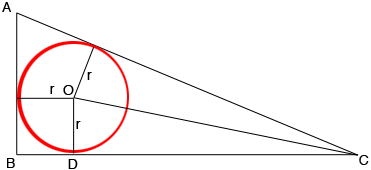SEARCH HOMEMath Central Quandaries & QueriesQuestion from akshaya, a student: A circle with centre O and radius r is inscribed in a right angled triangle ABC. If AB=5 cm, BC=12 cm and < B=90*, then find the value of r.Hi Akshaya,

I can help get you started.

First of all what does Pythagoras tell you is the length of the third side $CA$ of the triangle, $ABC?$In my diagram I drew a radius of the circle to each of the three points where the circle and triangle meet. Since the triangle side and the circle are tangent at these points the radius meets the triangle side at a right angle. What is the length of $BD?$ What is the length of $DC?$

PennyMath Central is supported by the University of Regina and The Pacific Institute for the Mathematical Sciences.